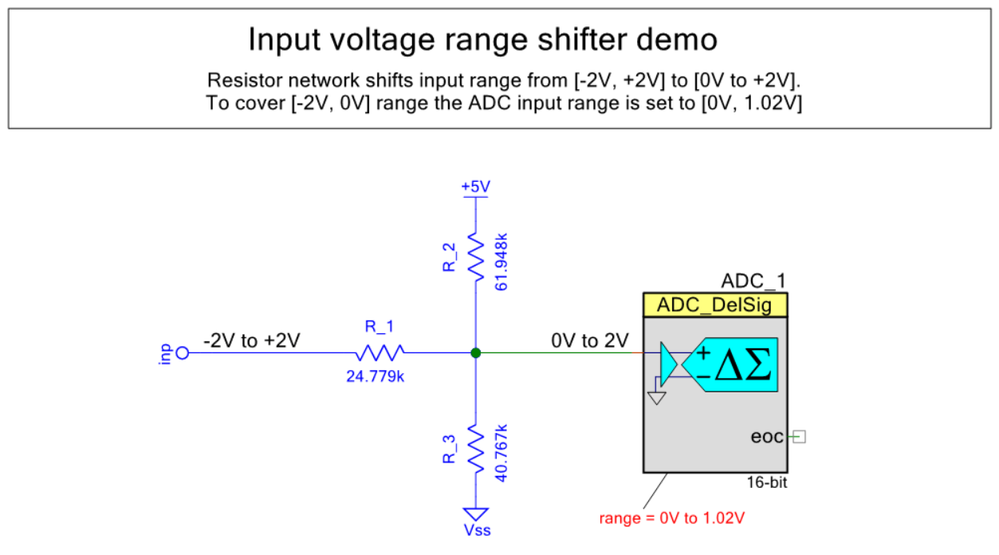PSoC™ 5, 3 & 1Level 2Level 2

Hi all,

Is it possible to read negative values with the ADC on the PSoC5 LP. If so how should it be configured?

I need to read values from -10mV to -1.5V.

1 SolutionLevel 9Level 9

JaRa,

One way to measure negative voltage is to use a resistor divider network as described by Aubrey Kagan:

Attached are a copy of the original paper, demo project, and Excel file to calculate resistor values. Note that there are only two binding equations and three resistor values, so the number of possible solutions is infinite. By selecting a starting values for R1,R2 and R3 it is possible to affect resulting solution. The Excel solver tends to slip into very large values (megaohms), which are not useful, to resolve this issue try to set some small starting values for R1-R3 (like 9).

Note that in order not to affect the resistor network, a Delsig_ADC is used, which has a buffer with very large input impedance. The ADC_SAR input impedance is rather small (~20k), affecting the measurement, so additional buffer Opamp is a must. Unfortunately the PSoC5 Opamp input impedance is not very high (~1M), so DelSig_ADC is preferred.

Once ADC voltage is measured, the input voltage can be calculated as:

Vin = ((RE1*RE2 + RE2*RE3 + RE1*RE3) * Vadc  -  RE1*RE3 * Vdd) / (RE2*RE3)

The calculated value depends on  the Vdd, which has to be stable for reproducible results.

/odissey1

Figure 1. Resistor network for shifting range from [-2V, +2V] into [0V, 2V] for ADC conversion. Note that ADC range is set to only half of that range [0V, 1.024V] to cover only negative portion of the input range.Resistor network R1-R3 to shift input range from [-2V, +2V] into [0V-2V] range. Note that ADC range is set to only half range [0,V 1.02V] to cover only negative input voltages.

Figure 2. Use Data->Solver to obtain resistor values (R1,R2,R3).Using Excel spreadsheet solver to obtain R1,R2,R3

13 Replies